## Combustion efficiency

The combustion efficiency ηf describes the sensible heat yield during combustion of a fuel. It is determined by calculating the thermal losses qA in the flue gas with reference to the ambient temperature level. Unburnt components of the fuel are not taken into account for oil and gas combustion since in practice they must not occur on a relevant scale.

The combustion efficiency is based on the net calorific value of a fuel and is calculated by deducting the flue gas losses from the maximum achievable 100%.Formula for calculating the combustion efficiency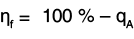Excess air

The excess air is the ratio of actual quantity of air supplied to the required stoichiometric quantity of air.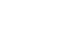The simplified equation for conversion of the flue gas oxygen content only applies for a flue gas/air ratio of ~ 1.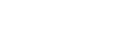Fig. "Correlation between oxygen content in dry flue gas, excess air and Siegert factor"

 λ Excess air ṁL Actual heat ṁL, st Stoichiometric heat O2 Oxygen [% by vol.]

To calculate the flue gas loss, the percentage of CO2 or O2 in the flue gas and temperature differential between the flue gas temperature and ambient temperature are determined. The maximum CO2 percentages in the flue gas, which depend on the fuel used in each case, and the Siegert factor f, which depends on the measured O2 content, are also required.Formula for calculating the flue gas loss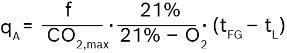qA Flue gas loss with reference to the combustion output and the lower net calorific value [%] f SSiegert factor, linear dependency on excess air λ [bar] CO2,max Maximum CO2 value in dry flue gas [% by vol.] O2 Measured oxygen content in dry flue gas [% by vol.] tFG Measured flue gas temperature [°C] tL Reference and combustion air temperature in accordance with EN 12953 part 11 constant 25°C

If only the CO2 value in the dry flue gas is measured, the following conversion applies:Formula for calculating the residual oxygen content from the CO2 value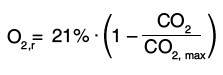O2,r Calculated oxygen content in dry flue gas [% by vol.] CO2 Measured CO2 value in dry flue gas [% by vol.] CO2,max Maximum CO2 value in dry flue gas [% by vol.]
 Siegert factor Siegert factor Fuel CO2,max f1 = f (O2 = 0 %) f2 = f (O2 = 5 %) Natural gas L 11.67 % 0.4792 0.4530 Natural gas H 11.94 % 0.4731 0.4469 Fuel oil EL 15.31 % 0.4535 0.4342 Fuel oil SA 16.02 % 0.4570 0.4389 Propane 13.69 % 0.4575 0.4352 Propane-Butane 13.78 % 0.4570 0.4349 Butane 13.99 % 0,.563 0.4346 Natural gas GZ35 11.12 % 0.4871 0.4611 Natural gas GZ41.5 11.67 % 0.4604 0.4358 Natural gas GZ50 11.67 % 0.4835 0.4569 Medium fuel oil HL Schwechat 15.72 % 0.4534 0.4348 Medium fuel oil CLU 3 16.11 % 0.4458 0.4285

Siegert factors of various fuelsCalculation of Siegert factor for any given oxygen content in dry flue gas O2: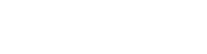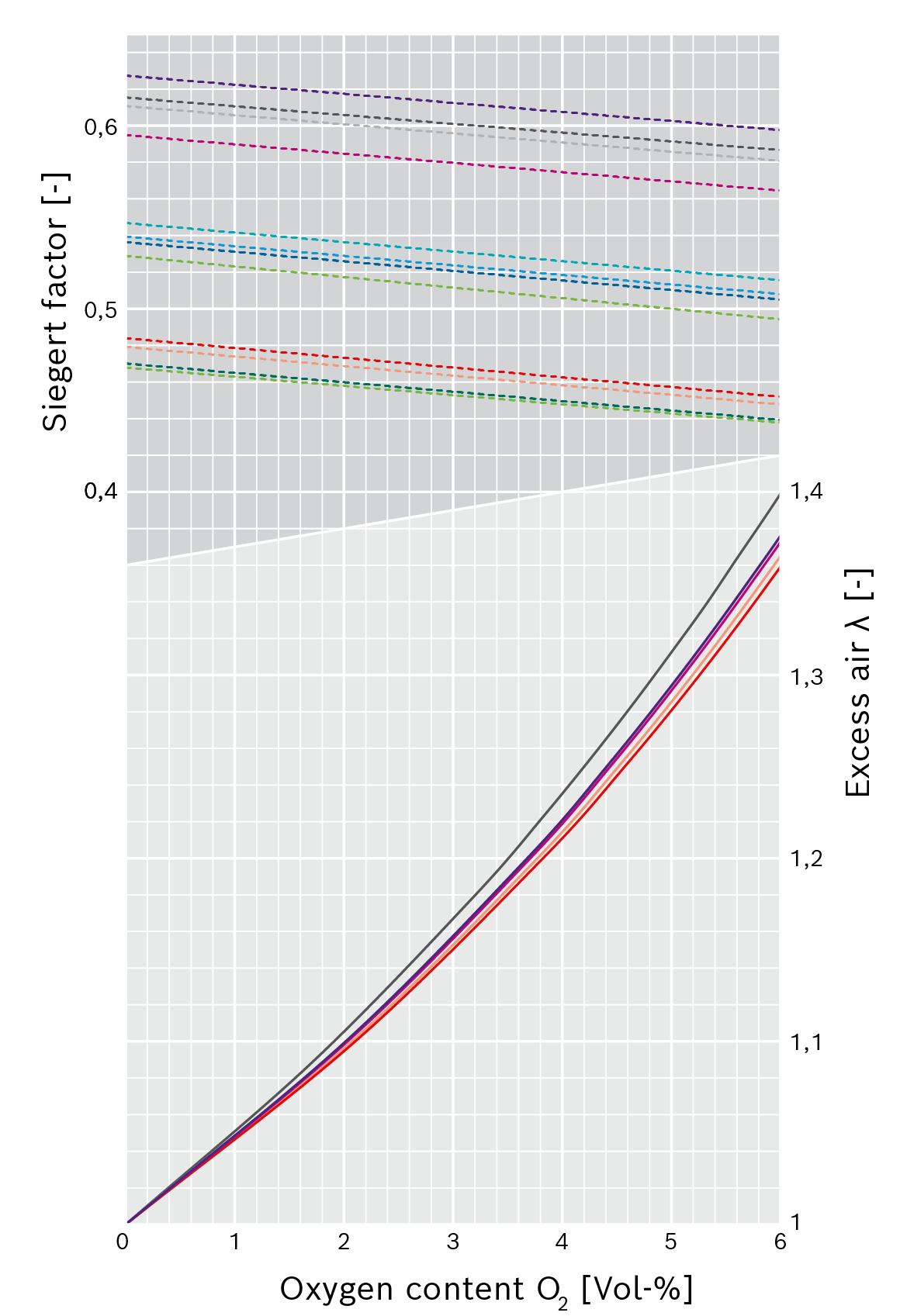Correlation between oxygen content in dry flue gas, excess air and Siegert factor21 % (21 % – O2)Natural gas LNatural gas HFuel oil ELFuel oil SANatural gas LNatural gas HFuel oil ELFuel oil SAPropanePropane-ButaneButaneNatural gas GZ35Natural gas GZ41.5Natural gas GZ50Medium fuel oil HL SchwechatMedium fuel oil CLU 3

Notes:

• For excess air: natural gas GZ 41.5/50, propane, butane, propane-butane coincides virtually exactly with natural gas L and is therefore not shown.
• Medium fuel oil CLU 3 and medium fuel oil HL Schwechat are between the curves of fuel oil EL and SA, and are therefore not shown.

The combustion efficiency increases starting from the full load until roughly 35% partial load with a boiler system. The excess air and therefore the CO2 content measured in the dry flue gas increases only slightly while the flue gas temperature falls due to more efficient utilisation of the heating surface in the boiler. At a partial load of <35% the greater amount of excess air which is necessary prevails and the combustion efficiency falls again.

The combustion efficiency is determined during emission measurement by the chimney sweep or customer service, for example. Heat losses due to radiation and conduction on the surface of the boiler are not taken into consideration in this case.

The relationship between combustion efficiency and flue gas temperature with varying excess air is shown in the following diagram for the fuel natural gas H. The higher the flue gas temperature the lower the efficiency.

The diagram also clearly shows that the increase in efficiency is particularly high with less excess air, i.e. low λ values, especially with high flue gas temperatures.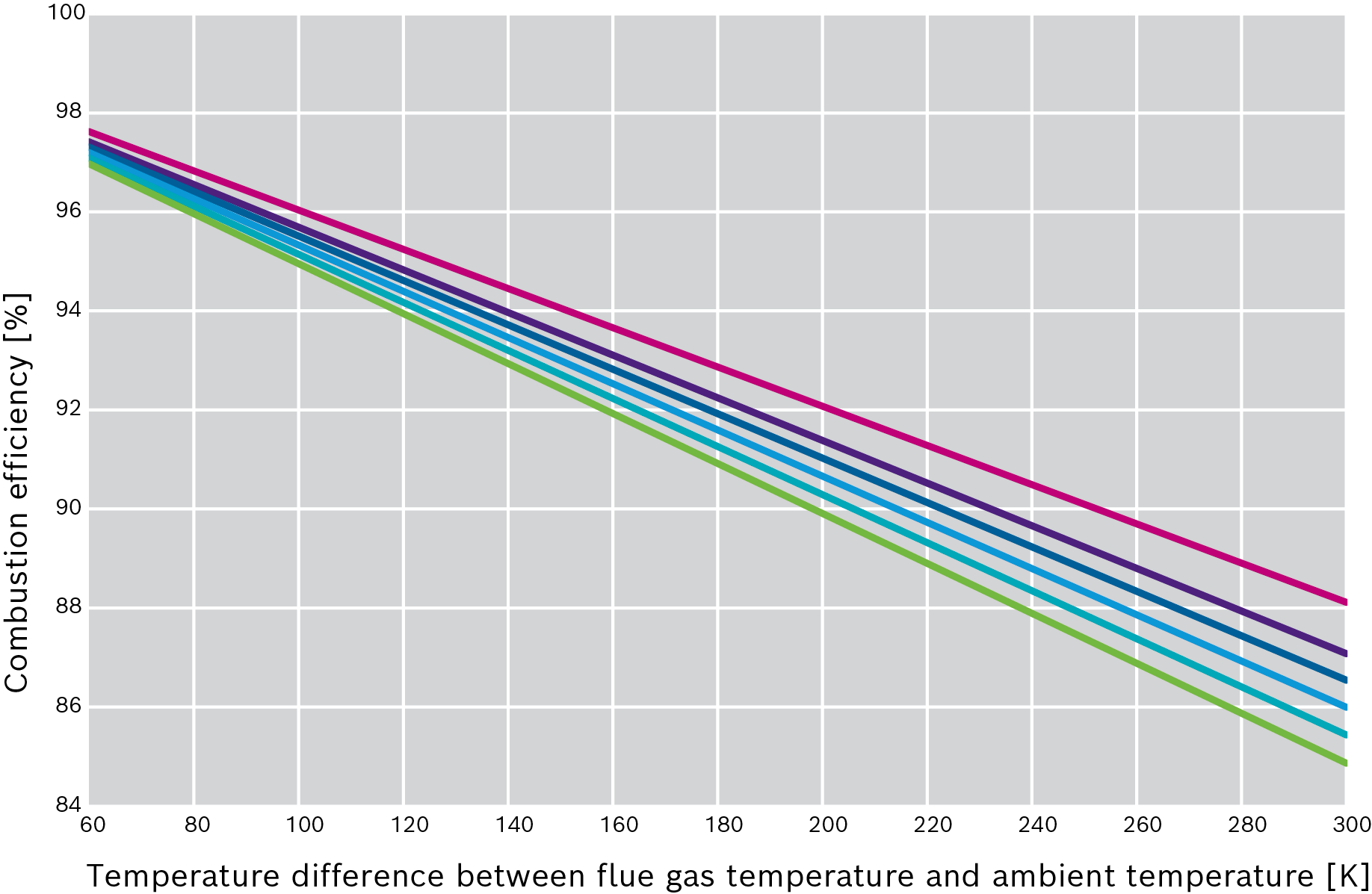Efficiency curve with reference to excess air λ without condensation, using natural gas H as exampleλ = 1 (O2 = 0 %)λ = 1.1 (O2 = 2.14 %)λ = 1.15 (O2 = 3.09 %)λ = 1.2 (O2 = 3.96 %)λ = 1.25 (O2 = 4.77 %)λ = 1.3 (O2 = 5.52 %)